plot.riskyr is a method that allows to generate different plot types from a "riskyr" object.

# S3 method for riskyr
plot(x = NULL, type = "prism", ...)

## Arguments

x An object of class "riskyr", usually a result of a call to riskyr. Pre-defined scenarios are also of type "riskyr". The type of plot to be generated. The following plot types are currently available: type = "prism" or type = "net" or type = "tree": Risk information is plotted in a network diagram of frequencies and probabilities (default). See plot_prism for further options. type = "tab" or type = "ftab": Risk information is plotted as a 2-by-2 frequency or contingency table. See plot_tab for further options. type = "area" or type = "mosaic": Risk information is plotted as a mosaic plot (scaled area). See plot_area for further options. type = "bar" or type = "fbar": Risk information is plotted as a bar chart. See plot_bar for further options. type = "icons" or type = "iconarray": The underlying population is plotted as an array of icons. See plot_icons for further options. type = "curve" or type = "curves": Draws curves of selected values (including PPV, NPV) See plot_curve for further options. type = "plane" or type = "planes": Draws a 3D-plane of selected values (e.g., predictive values PPV or NPV) See plot_plane for further options. Additional parameters to be passed to the underlying plotting functions.

## Details

plot.riskyr also uses the text settings specified in the "riskyr" object.

riskyr initializes a riskyr scenario.

Other visualization functions: plot_area, plot_bar, plot_curve, plot_fnet, plot_icons, plot_mosaic, plot_plane, plot_prism, plot_tab, plot_tree

Other riskyr scenario functions: read_popu, riskyr, summary.riskyr

## Examples

# Select a scenario (from list of scenarios):
s1 <- scenarios\$n1  # select scenario 1 from scenarios
plot(s1)  # default plot (type = "prism")
# Plot types currently available:
plot(s1, type = "prism")                # prism/network diagram (default)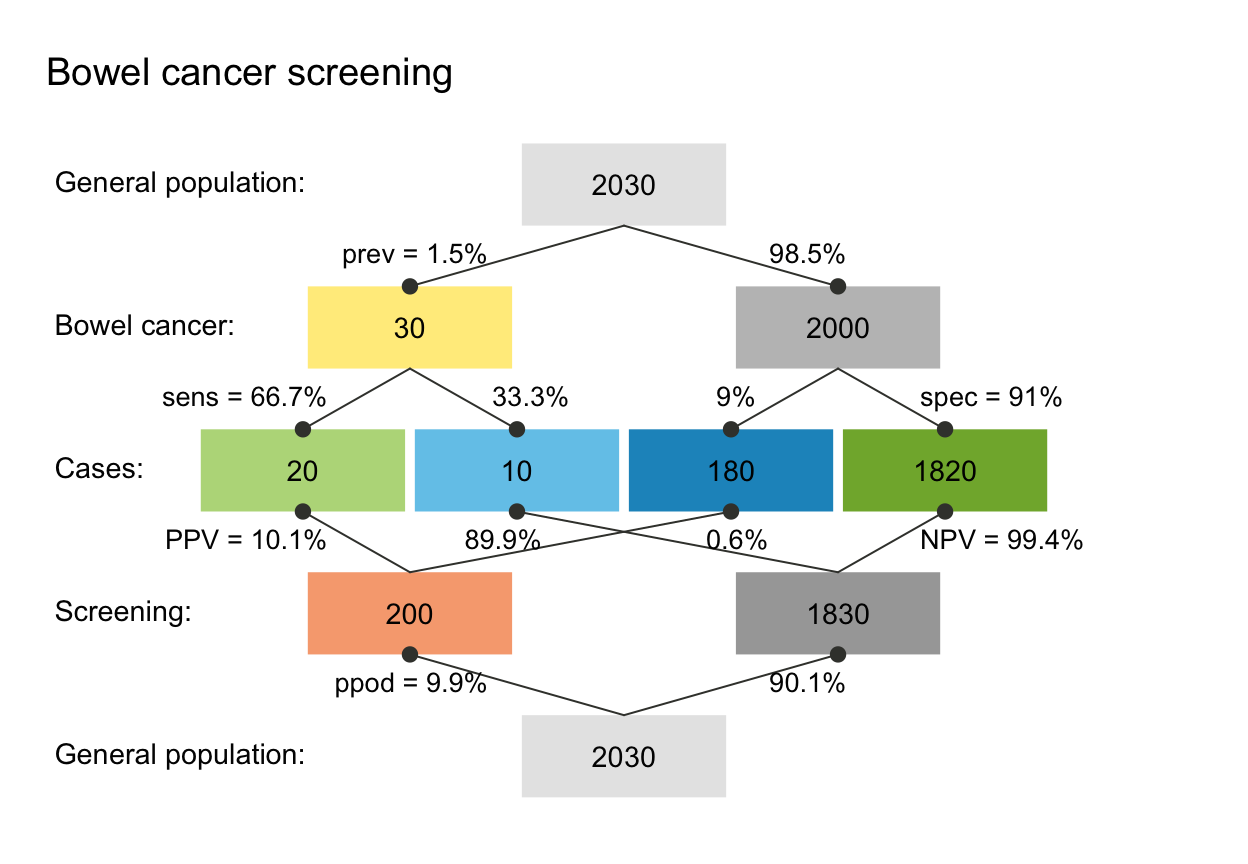plot(s1, type = "tree", by = "cd")      # tree diagram (only 1 perspective)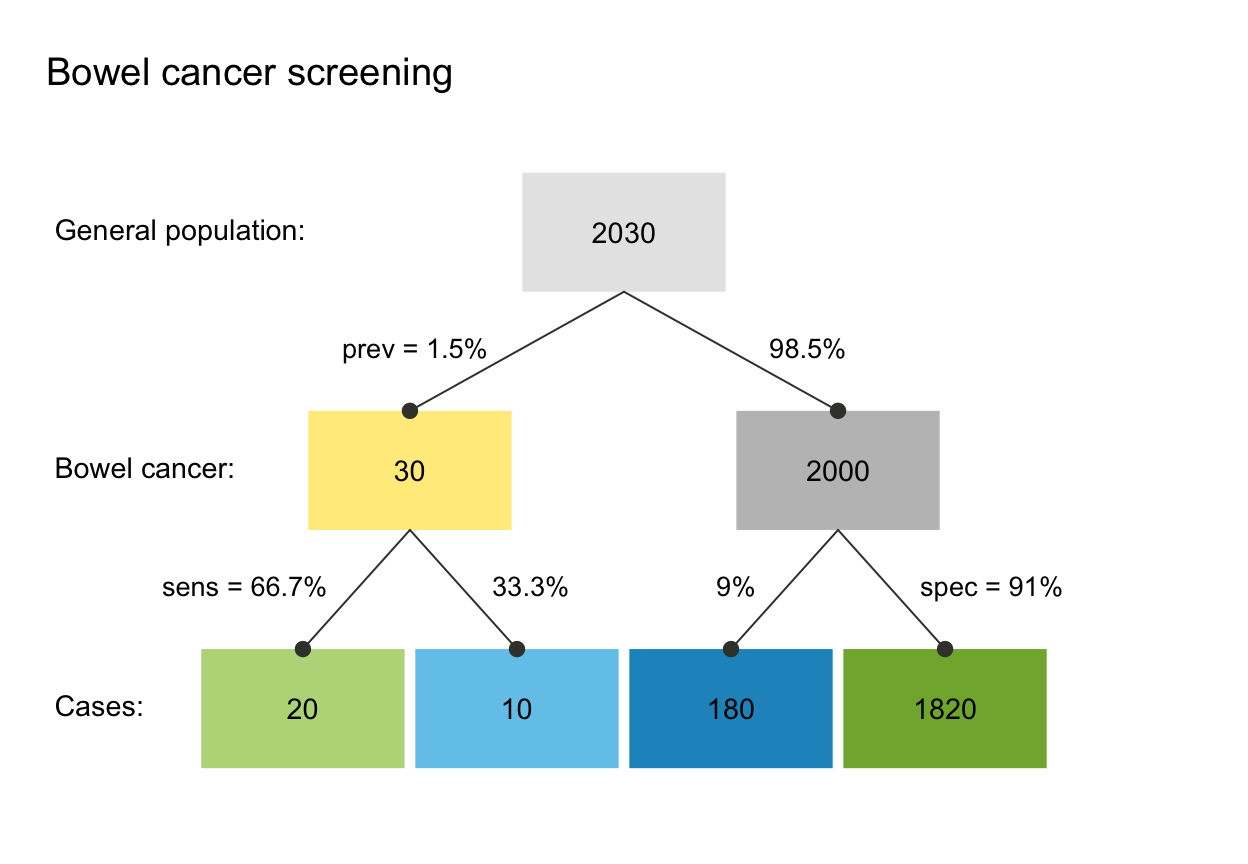plot(s1, type = "area")                 # area/mosaic plot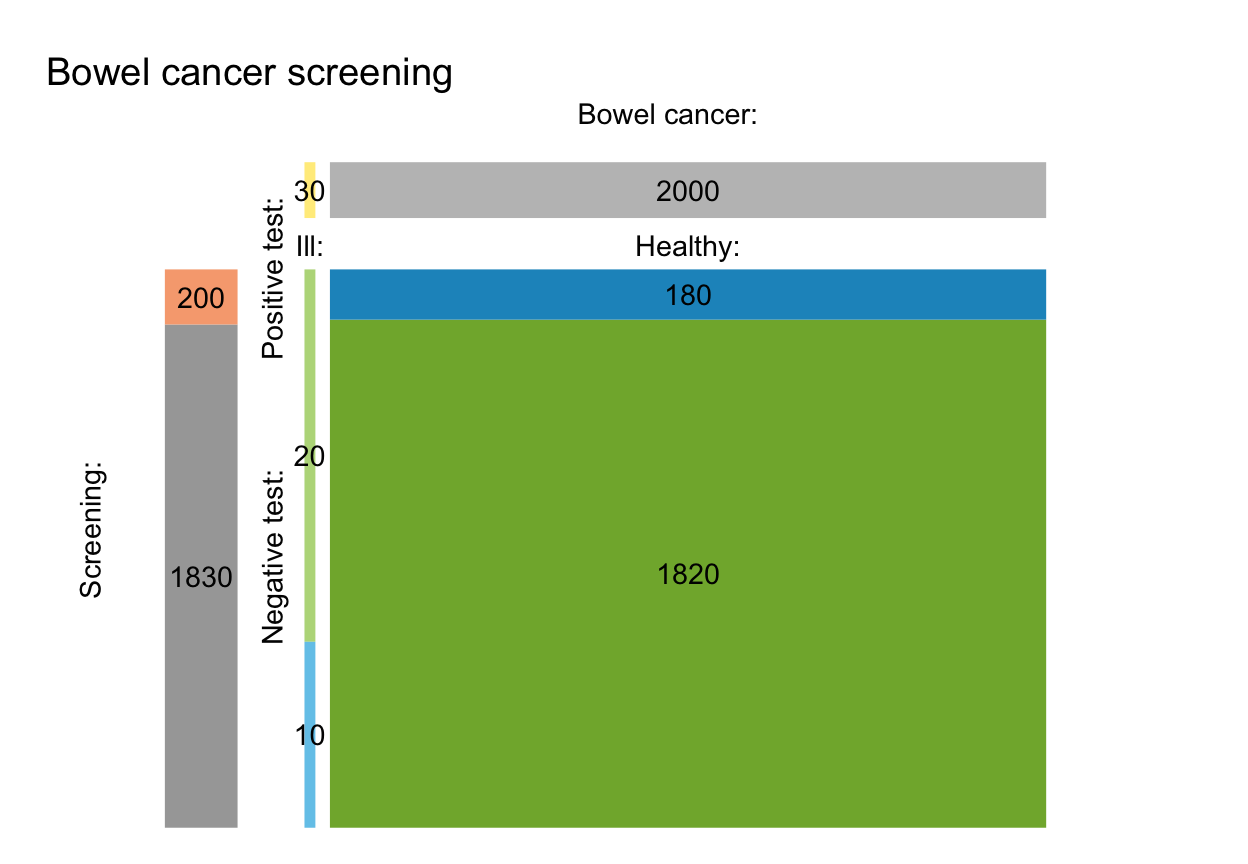plot(s1, type = "tab")                  # 2x2 frequency/contingency table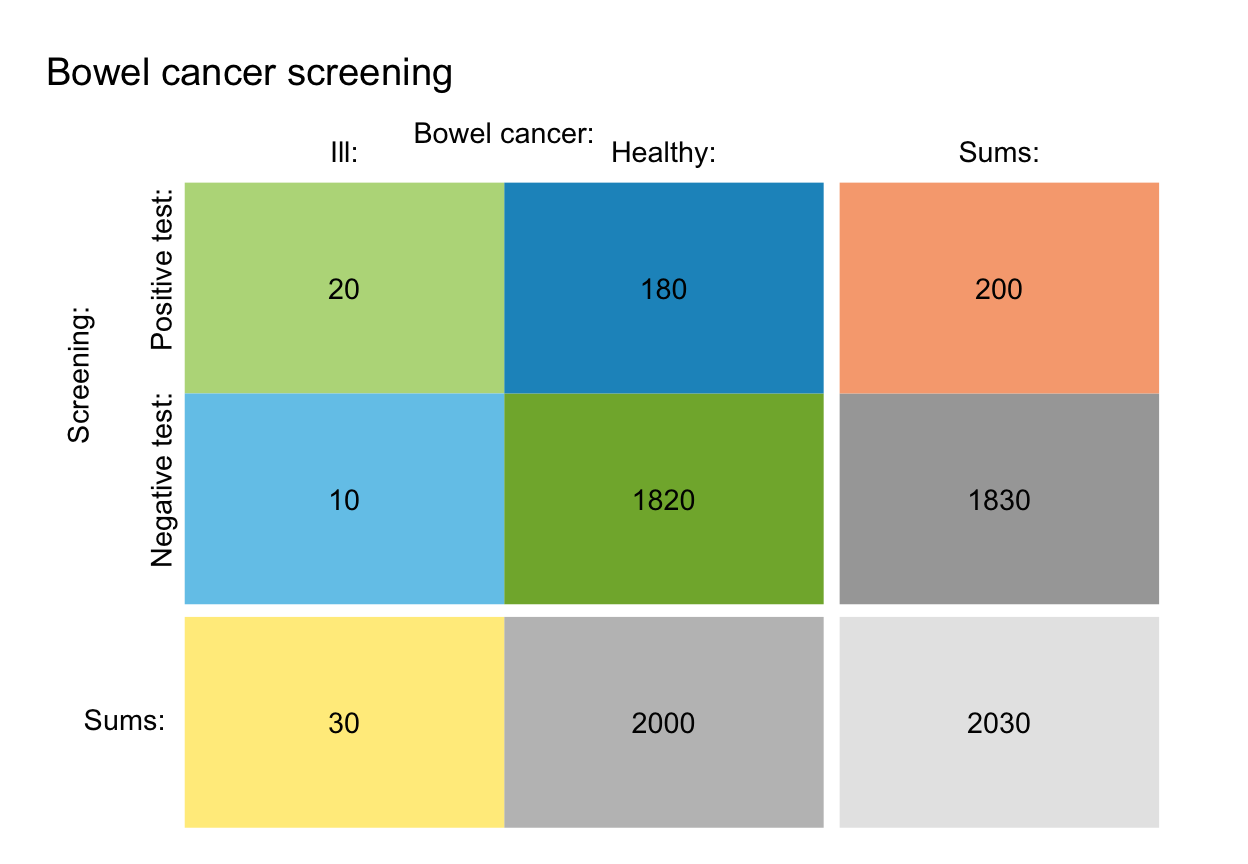plot(s1, type = "bar", dir = 2)         # bar plot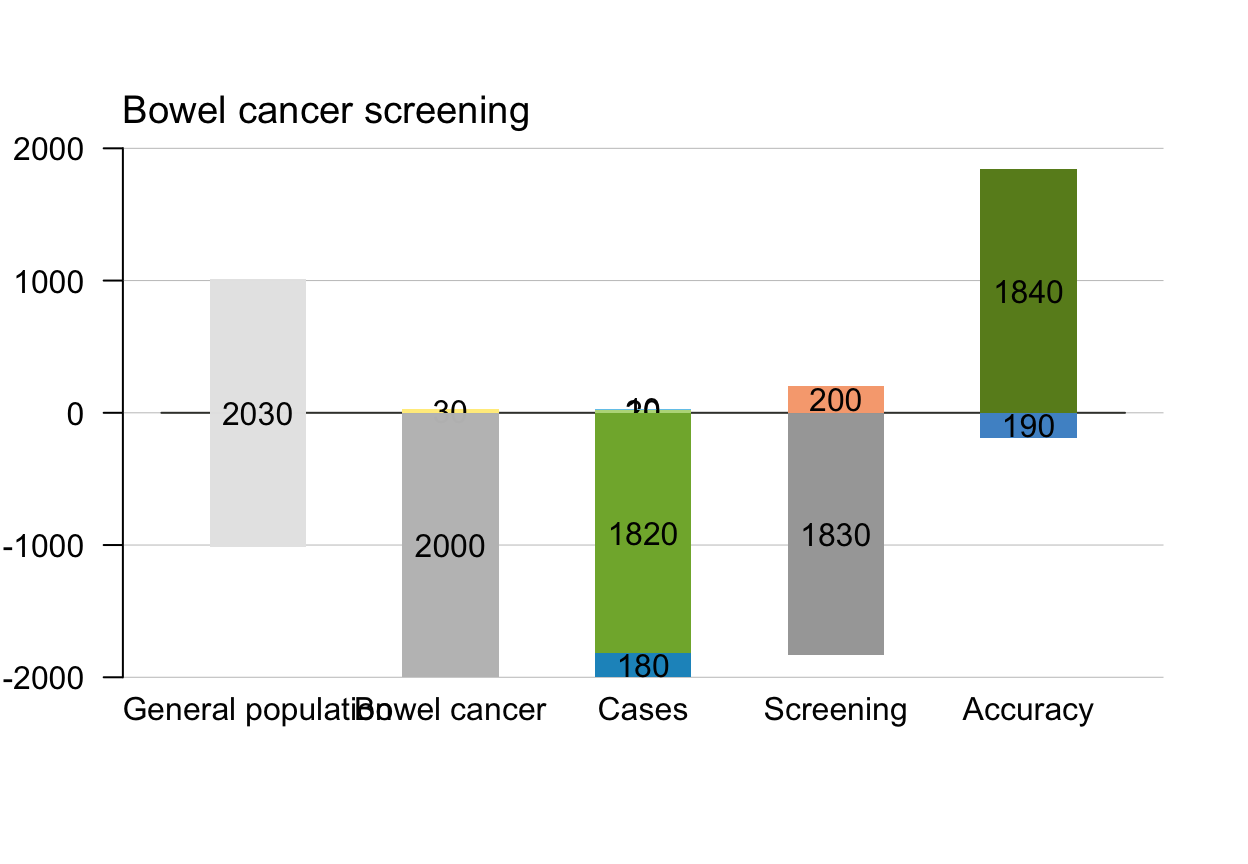plot(s1, type = "icons")                # icon array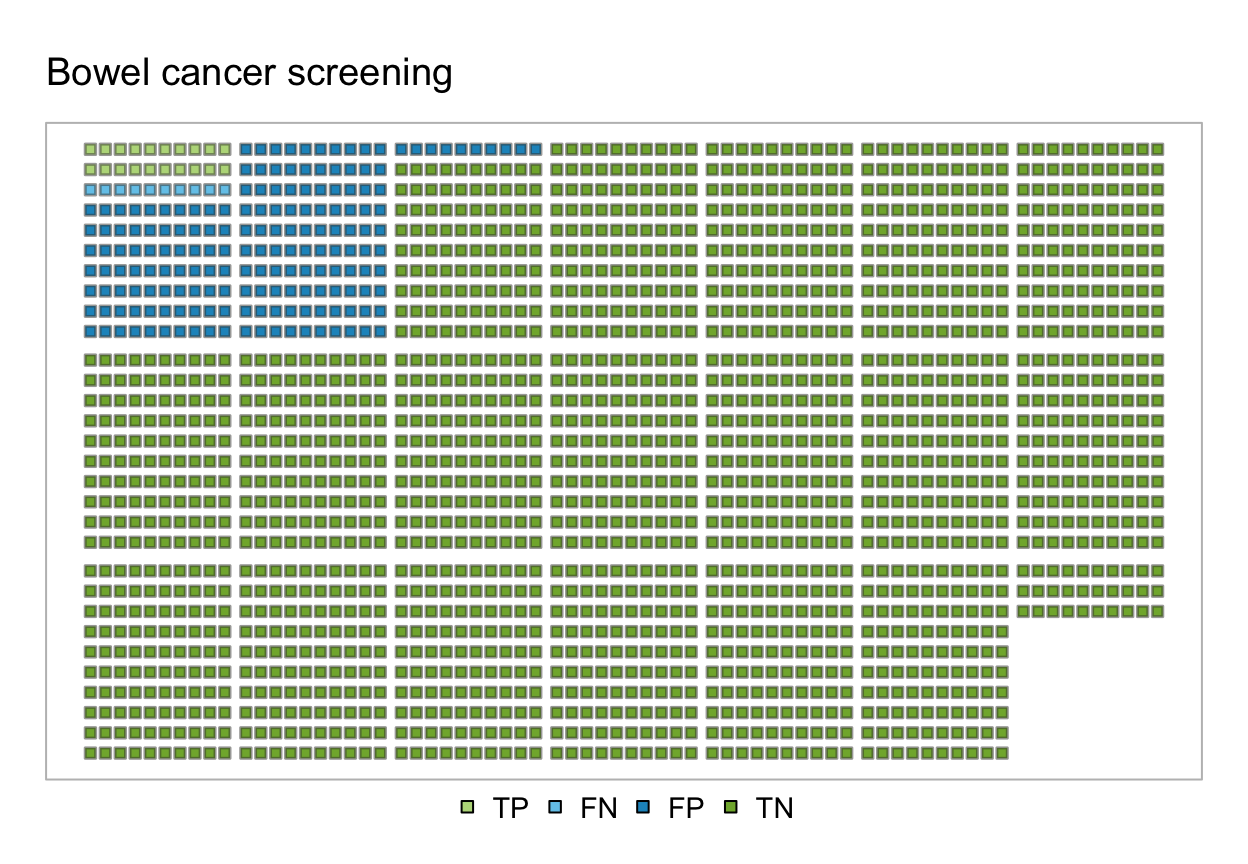plot(s1, type = "curve", what = "all")  # curves as fn. of prev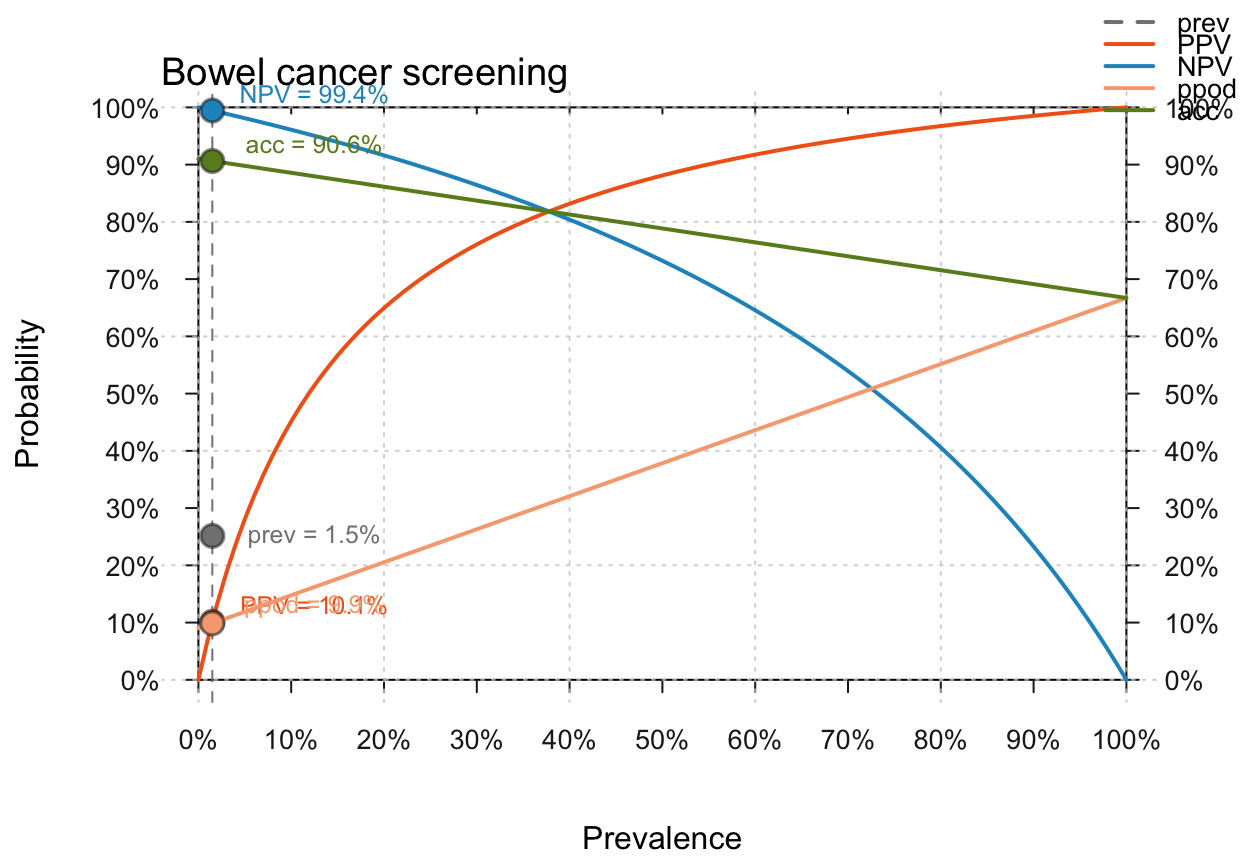plot(s1, type = "plane", what = "NPV")  # plane as function of sens & spec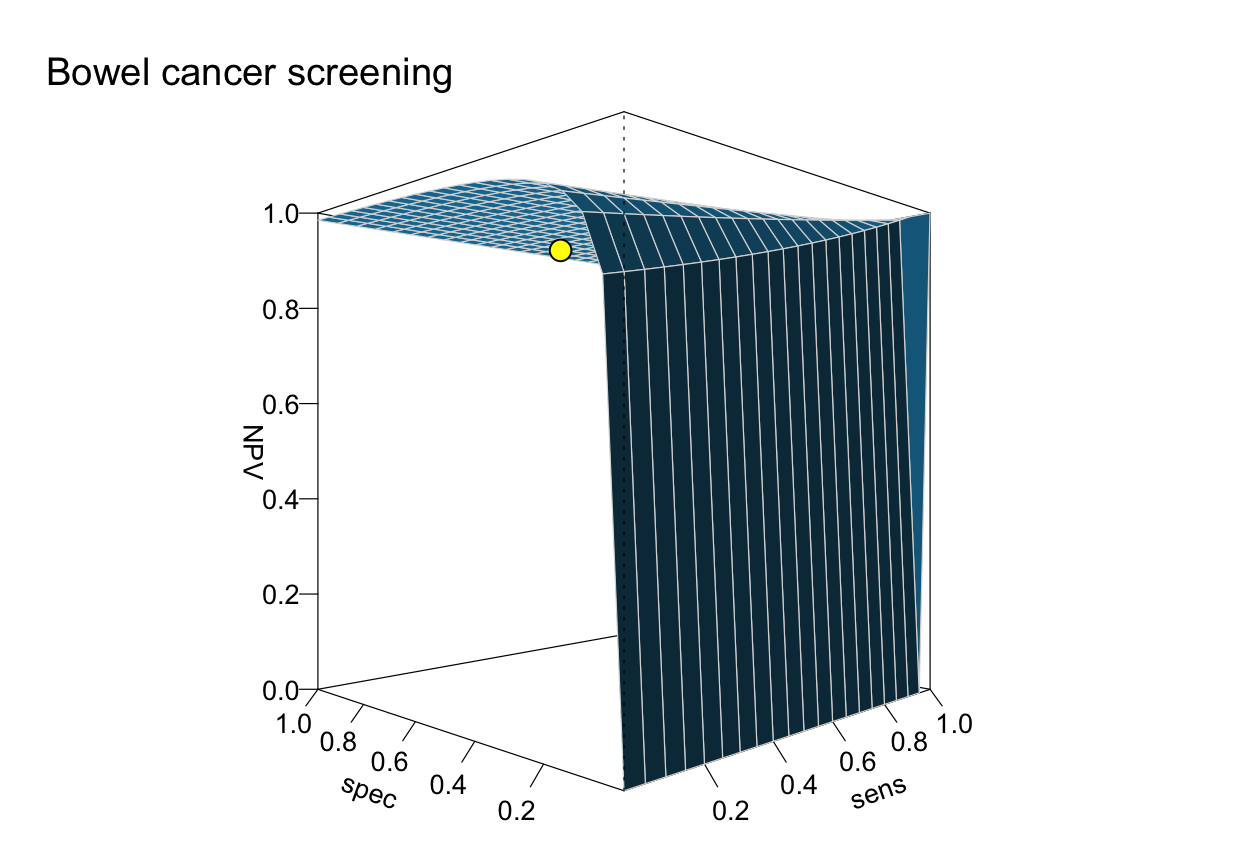plot(s1, type = "default")              # unknown type: use default plot#> Unknown plot type (in plot.riskyr): Using type = 'prism'.